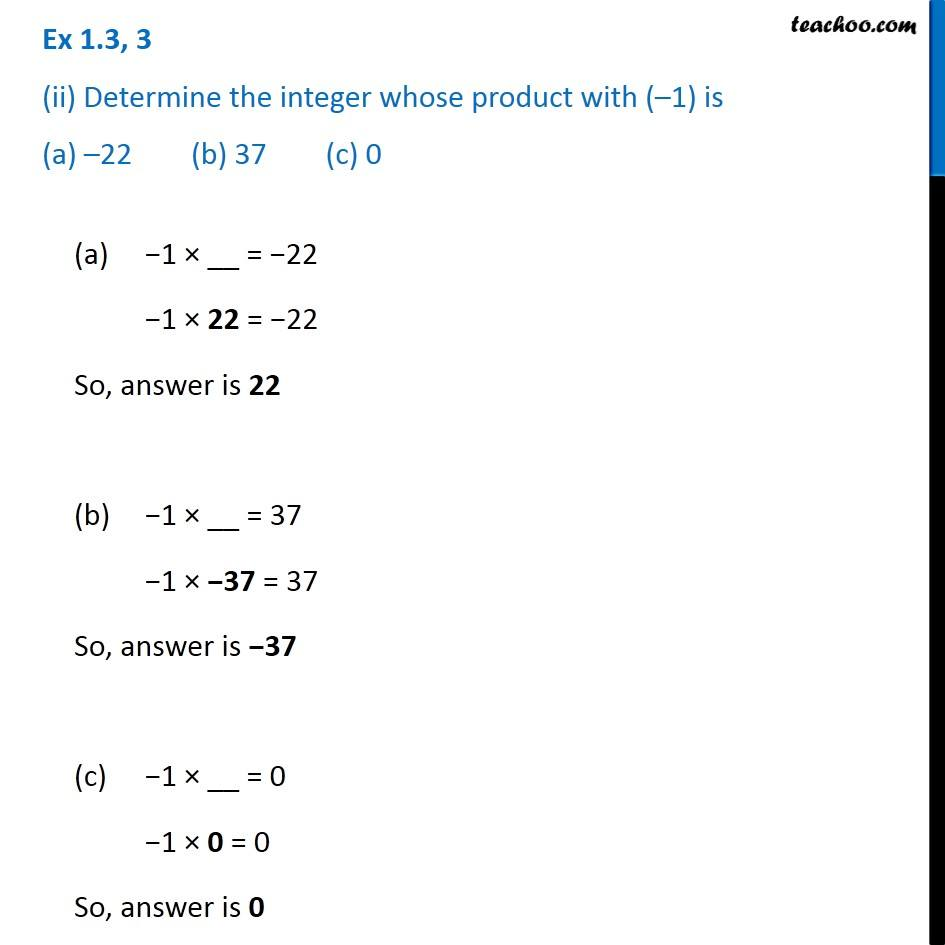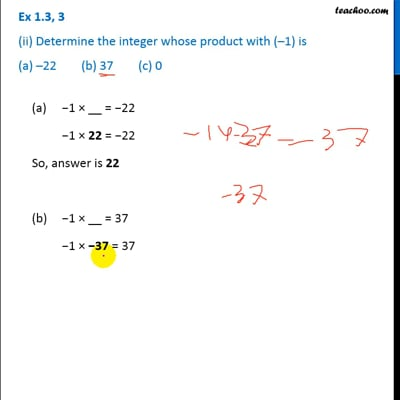Ex 1.3

Chapter 1 Class 7 Integers
Serial order wiseThis video is only available for Teachoo black users

Introducing your new favourite teacher - Teachoo Black, at only ₹83 per month

### Transcript

Ex 1.3, 3 (ii) Determine the integer whose product with (–1) is (a) –22 (b) 37 (c) 0 (a) −1 × __ = −22 −1 × 22 = −22 So, answer is 22 (b) −1 × __ = 37 −1 × −37 = 37 So, answer is −37 (c) −1 × __ = 0 −1 × 0 = 0 So, answer is 0